# Selina Concise Mathematics Class 9 ICSE Solutions Indices (Exponents)

## Selina Concise Mathematics Class 9 ICSE Solutions Indices (Exponents)

APlusTopper.com provides step by step solutions for Selina Concise Mathematics Class 9 ICSE Solutions Chapter 7 Indices (Exponents). You can download the Selina Concise Mathematics ICSE Solutions for Class 9 with Free PDF download option. Selina Publishers Concise Mathematics for Class 9 ICSE Solutions all questions are solved and explained by expert mathematic teachers as per ICSE board guidelines.

Selina ICSE Solutions for Class 9 Maths Chapter 7 Indices (Exponents)

Exercise 7(A)

Solution 1: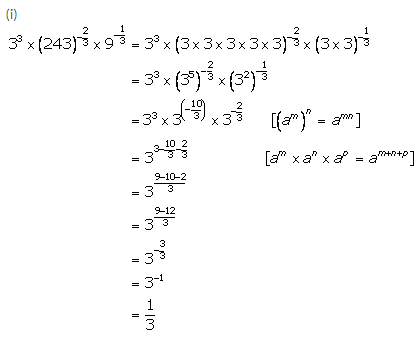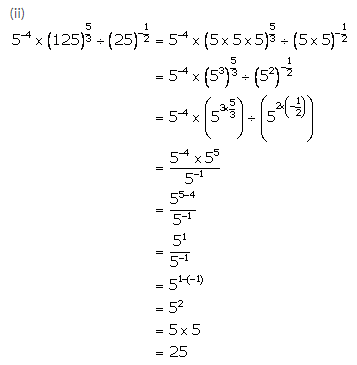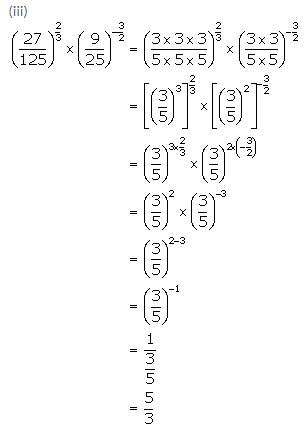Solution 2:Solution 3: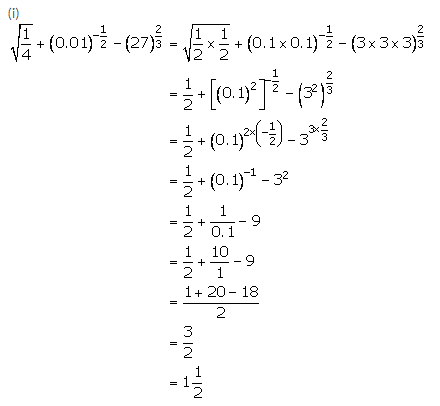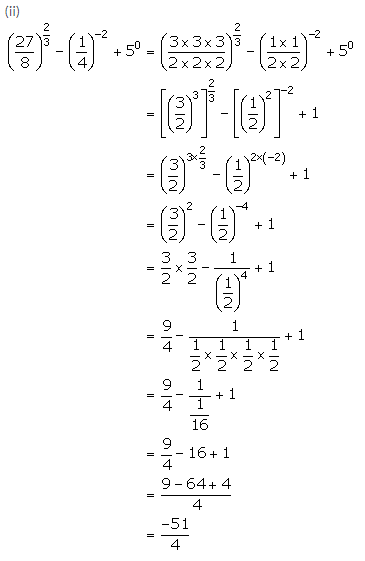Solution 4: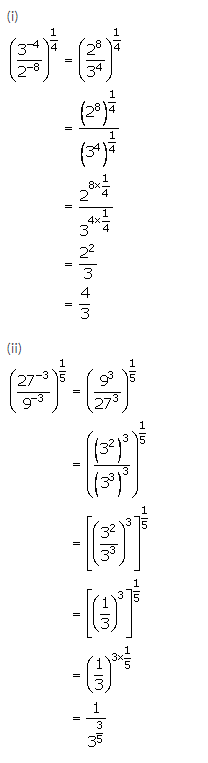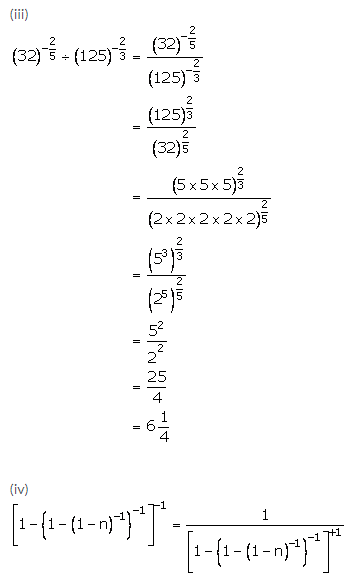Solution 5:Solution 6:Solution 7: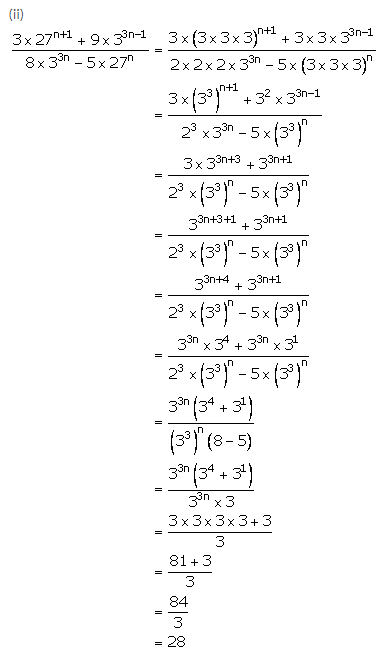Solution 8:Solution 9:Solution 10: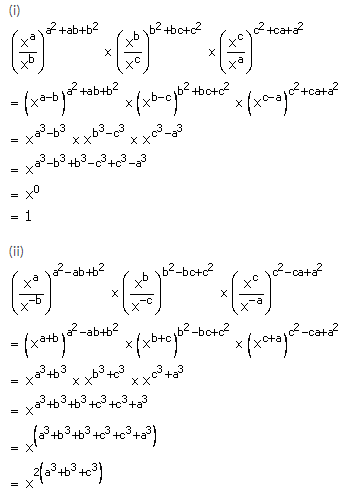Exercise 7(B)

Solution 1: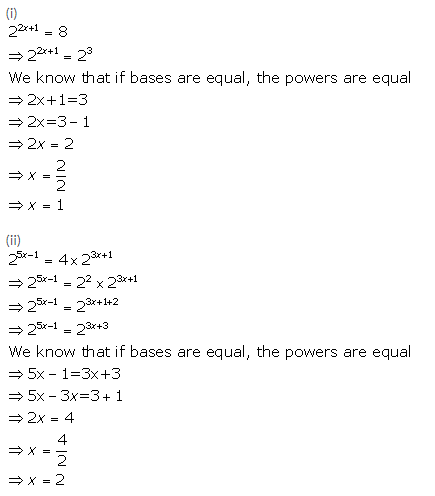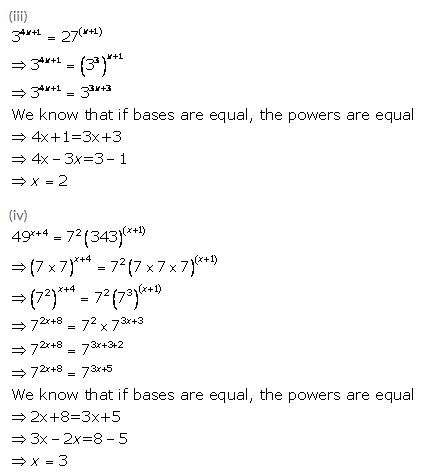Solution 2:Solution 3:Solution 4: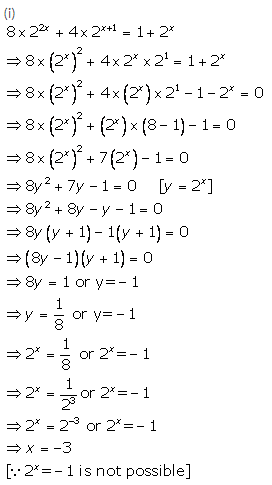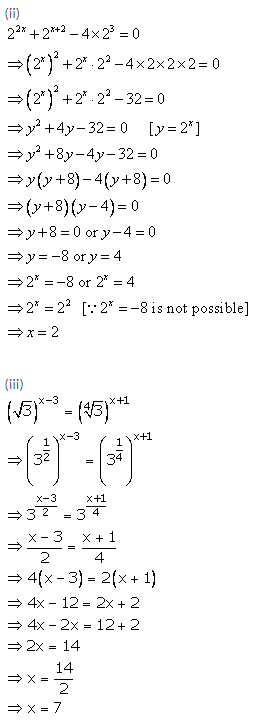Solution 5: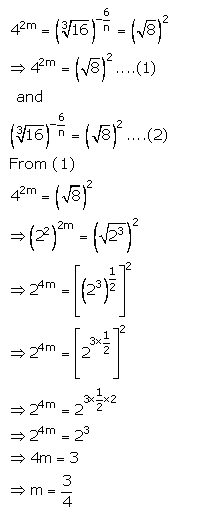Solution 6: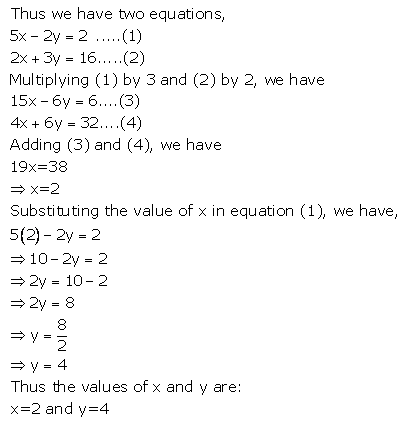Solution 7: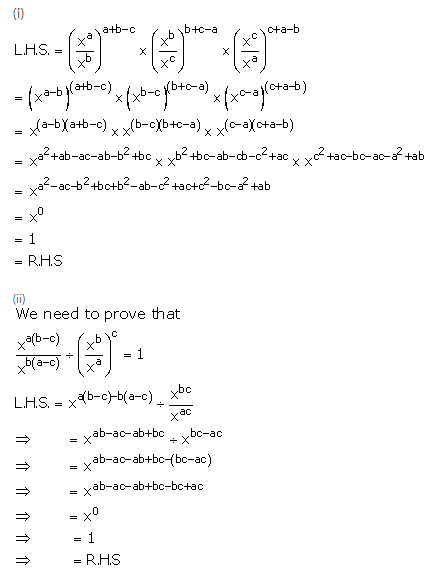Solution 8: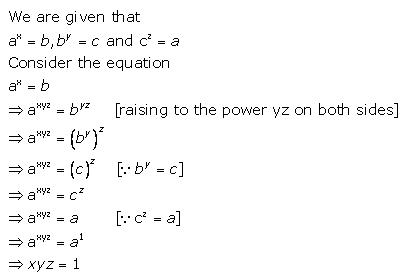Solution 9: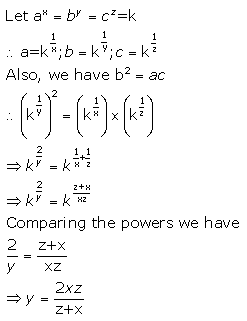Solution 10: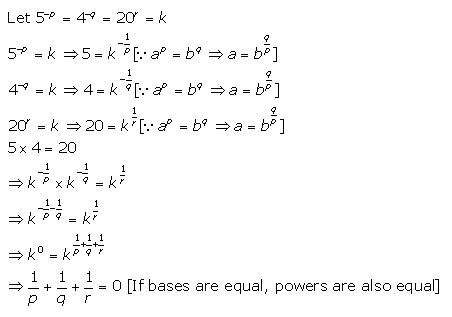Solution 11: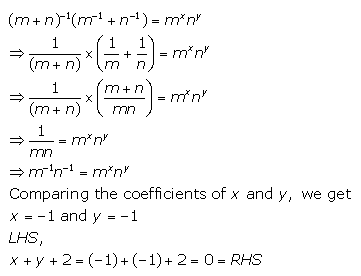Solution 12:Solution 13: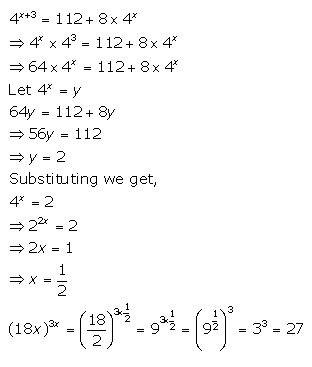Solution 14(i):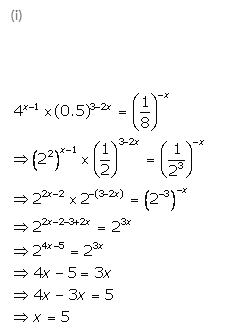Solution 14(ii):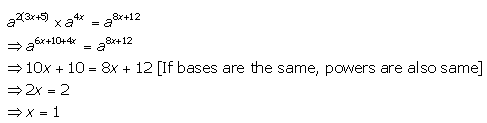Solution 14(iii):Solution 14(iv):Solution 14(v):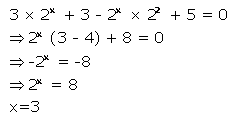Exercise 7(C)

Solution 1: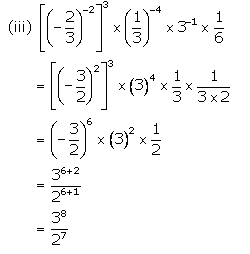Solution 2:Solution 3:Solution 4: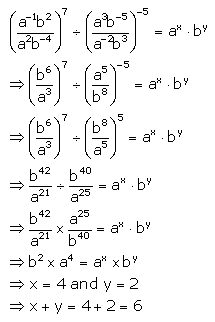Solution 5:Solution 6: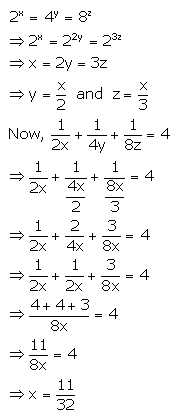Solution 7:Solution 8:Solution 9: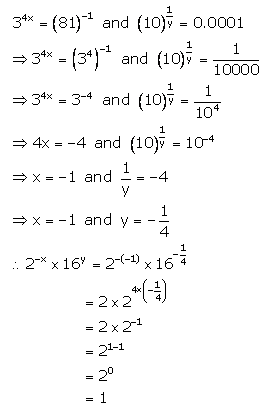Solution 10: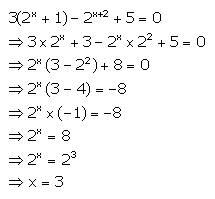Solution 11: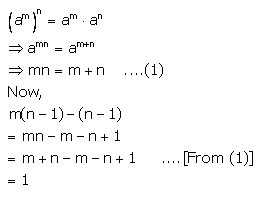Solution 12:More Resources for Selina Concise Class 9 ICSE Solutions